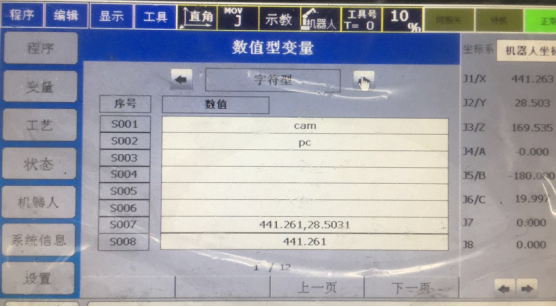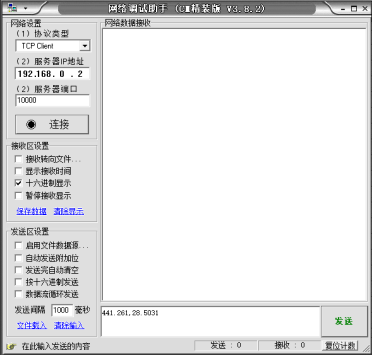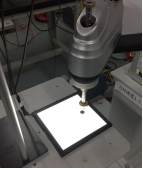## 10.1 標定原理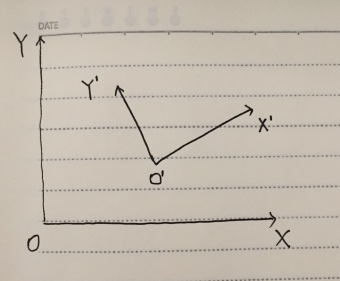（為了對應後面程式，所以座標名稱直接使用下文中的程式變數名）

imageP1P2.x = imageP2.x - imageP1.x

imageP1P2.y = imageP2.y - imageP1.y

robotP1P2.x = robotP2.x - robotP1.x

robotP1P2.y = robotP2.y - robotP1.y

factor = sqrt(robotP1P2.x*robotP1P2.x + robotP1P2.y*robotP1P2.y)/ sqrt(imageP1P2.x*imageP1P2.x + imageP1P2.y*imageP1P2.x)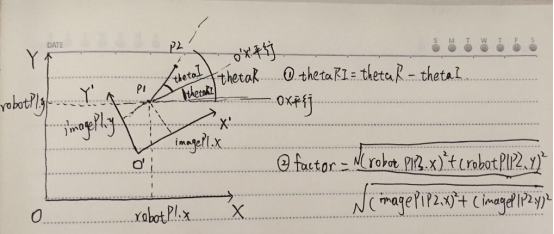thetaR = atan2((robotP2.y - robotP1.y) ,(robotP2.x - robotP1.x))

thetaI = atan2((imageP2.y - imageP1.y) , (imageP2.x - imageP1.x))

thetaRI = thetaR - thetaI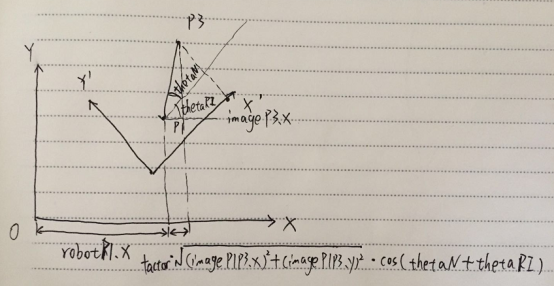imageP1P3.x = imageP3.x - imageP1.x

imageP1P3.y = imageP3.y - imageP1.y

robotP3.x = factor*sqrt(imageP1P3.x*imageP1P3.x + imageP1P3.y*imageP1P3.y)* cos(thetaN + thetaRI) + robotP1.x

robotP3.y = factor*sqrt(imageP1P3.x*imageP1P3.x + imageP1P3.y*imageP1P3.y)* sin(thetaN + thetaRI) + robotP1.y

## 10.2 標定程式實現

` `
1. `/*`

2. `機器人座標系與相機座標系轉換程式`

3. `第一步：記錄A點的機器人座標系下座標以及影象座標系下座標，記錄B點的機器人座標系下座標以及影象座標系下座標；`

4. `第二步：計算得到現實距離與影象畫素之間的比例係數，以及兩座標系的偏轉角；`

5. `前兩步完成後，即完成了標定過程，接下來就可以根據一個新的點的影象座標系下的座標，求得該點的機器人座標點；`

6. `第三步：輸入一個影象座標系下的點，程式自動得到機器人座標下的點。`

7. `*/`

8.
9. `#include<iostream>`

10. `#include<cmath>`

11. `using namespace std;`

12.
13. `struct position {`

14. `double x;`

15. `double y;`

16. `};`

17.
18. `struct vector {`

19. `double x;`

20. `double y;`

21. `};`

22.
23. `int main()`

24. `{`

25. `//1 input the positions in the camera coodinate and robot coodinate`

26. `position imageP1, imageP2, robotP1, robotP2;`

27. `cout << "請輸入相機座標系下的座標" << endl;`

28. `cin >> imageP1.x >> imageP1.y >> imageP2.x >> imageP2.y;`

29. `cout << "請輸入機器人座標系下的座標" << endl;`

30. `cin >> robotP1.x >> robotP1.y >> robotP2.x >> robotP2.y;`

31.
32. `//2 caculate the factor and tangle`

33. `vector imageP1P2, robotP1P2;`

34. `imageP1P2.x = imageP2.x - imageP1.x;`

35. `imageP1P2.y = imageP2.y - imageP1.y;`

36. `robotP1P2.x = robotP2.x - robotP1.x;`

37. `robotP1P2.y = robotP2.y - robotP1.y;`

38.
39. `double factor, thetaI, thetaR, thetaRI;`

40. `factor = sqrt(robotP1P2.x*robotP1P2.x + robotP1P2.y*robotP1P2.y)/ sqrt(imageP1P2.x*imageP1P2.x + imageP1P2.y*imageP1P2.x);`

41. `thetaR = atan2((robotP2.y - robotP1.y) ,(robotP2.x - robotP1.x));`

42. `thetaI = atan2((imageP2.y - imageP1.y) , (imageP2.x - imageP1.x));`

43. `thetaRI = thetaR - thetaI;`

44. `cout <<"兩座標系的弧度偏離角為"<< thetaRI << endl;`

45.
46.
47. `//3 input a new postion in the camera coodinate, computer will caculate the postion information in the robot coodinate`

48. `position imageP3,robotP3;`

49. `cout << "請輸入一個新的影象座標系點" << endl;`

50. `cin >> imageP3.x >> imageP3.y;`

51. `vector imageP1P3;`

52. `imageP1P3.x = imageP3.x - imageP1.x;`

53. `imageP1P3.y = imageP3.y - imageP1.y;`

54. `double thetaN;`

55. `thetaN = atan2(imageP1P3.y, imageP1P3.x);`

56.
57. `robotP3.x = factor*sqrt(imageP1P3.x*imageP1P3.x + imageP1P3.y*imageP1P3.y)* cos(thetaN + thetaRI) + robotP1.x;`

58. `robotP3.y = factor*sqrt(imageP1P3.x*imageP1P3.x + imageP1P3.y*imageP1P3.y)* sin(thetaN + thetaRI) + robotP1.y;`

59.
60. `cout << "機器人座標系下的該點座標為：" << endl;`

61. `cout << robotP3.x << "," << robotP3.y << endl;`

62.
63. `system("pause");`

64. `return 0;`

65. `}`

matlab計算程式如下：

` `
1. `clear; clc; close all;`

2.
3. `Ar = [411.428 16.614]; % point A in robot coordinate`

4. `Br = [403.211 -109.381]; % point B in robot coordinate`

5.
6. `Ai = [947 90]; % point A in image coordinate`

7. `Bi = [525 70]; % point B in image coordinate`

8.
9. `AiBi = Bi - Ai`

10. `ArBr = Br - Ar`

11.
12. `% calculate mm and pixel coefficient`

13. `MM_PER_PIXEL = norm(ArBr) / norm(AiBi)`

14.
15. `% swap image coordinate xi and yi`

16. `theta_i = atan2d( AiBi(1), AiBi(2) )`

17. `% theta_i = atan2d( AiBi(2), AiBi(1) )`

18. `theta_r = atan2d( ArBr(2), ArBr(1) )`

19.
20. `THETA_IR = theta_r - theta_i`

21.
22. `%%`

23. `Ni = [627 333]; % new point in image coordinate`

24. `AiNi = Ni - Ai;`

25. `theta_ni = atan2d(AiNi(1),AiNi(2));`

26.
27. `Nrx = MM_PER_PIXEL*norm(AiNi)*cosd(theta_ni+THETA_IR) + Ar(1);`

28. `Nry = MM_PER_PIXEL*norm(AiNi)*sind(theta_ni+THETA_IR) + Ar(2);`

29.
30. `Nr = [Nrx Nry]`

31.
32.
33.
34. `% robot = [428.8647 59.8150]`

35. `% z = 227.8875`

36.

## 10.3 工業智慧相機與SCARA機械手座標系的標定例項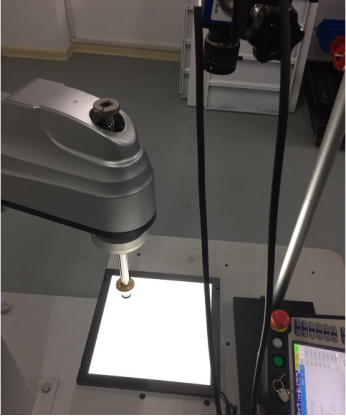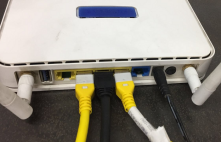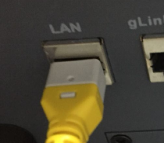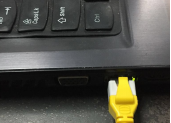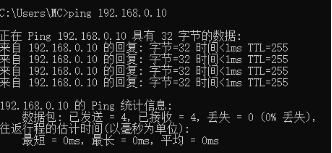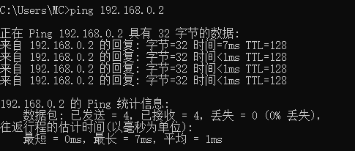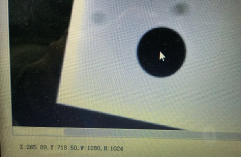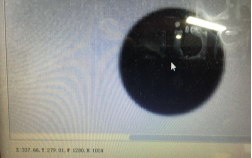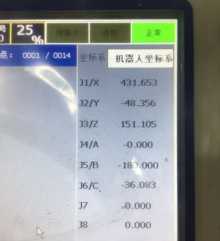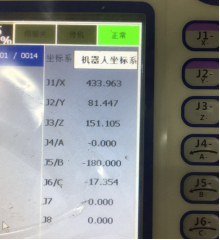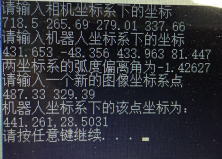NOP MOVJ P87 V=20% BL=0 VBL=0 SET STR=7 SOCKCLOSE str2 SOCKOPEN str2 type=SERVER TIMER T=10000ms SOCKRECV str2 str7 I1 STRSPLIT DELIM=, str7 str8 N=1 STRSPLIT DELIM=, str7 str9 N=2 STR2REAL str8 R8 STR2REAL str9 R9 SETE P88.1 R8 SETE P88.2 R9 MOVJ P88 V=20% BL=0 VBL=0 NOP